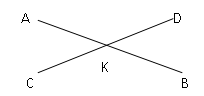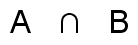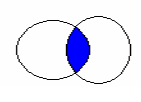Home Math Calculators Math  Flashcards Math Games Math  Homework Math Lessons Math Terms Math Worksheets Math Tools
You Are In Math Glossary of Terms

# Intersection

(A) In Geometry, an intersection is a single point where two lines or curves meet or cross each other.In the figure above, point K is the intersection of line segments AB and CD.

Another way it may be said is that the line segment CD intersects AB at point K.

(B)In set theory,an intersection of two sets A and B is the set that contains all elements of A that also belong to B (or equivalently, all elements of B that also belong to A), but no other elements.